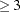The PRINCOMP Procedure
 ODS Graphics

To request graphics with PROC PRINCOMP, you must first enable ODS Graphics by specifying the ods graphics on statement. See Chapter 21, Statistical Graphics Using ODS, for more information. Some graphs are produced by default; other graphs are produced by using statements and options. You can reference every graph produced through ODS Graphics with a name. The names of the graphs that PROC PRINCOMP generates are listed in Table 69.3, along with the required statements and options.

Table 69.3 ODS Graphics Produced by PROC PRINCOMP

ODS Graph Name

Plot Description

Statement and Option

PaintedScorePlot

Score plot of component 3 versus component 2, painted by component 1

PLOTS=SCORE when number of variablesPatternPlot

Component pattern plot

PLOTS=PATTERN

PatternProfilePlot

Component pattern profile plot

PLOTS=PATTERNPROFILE

ScoreMatrixPlot

Matrix plot of component scores

PLOTS=MATRIX

ScorePlot

Component score plot

PLOTS=SCORE

ScreePlot

Scree and variance plots

default and PLOTS=SCREE

VariancePlot

Variance proportion explained plot

PLOTS=SCREE(UNPACKPANEL)Previous Page | Next Page | Top of Page